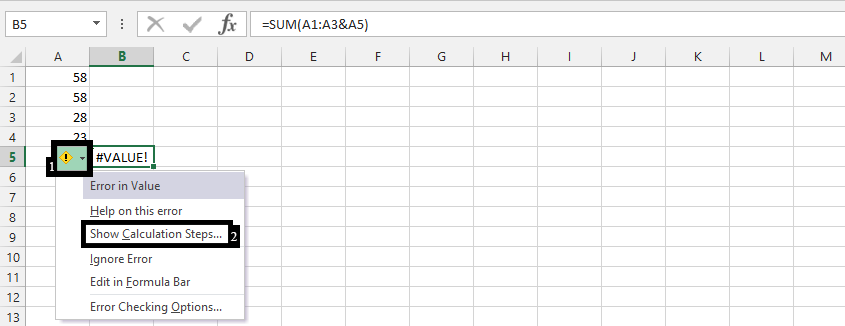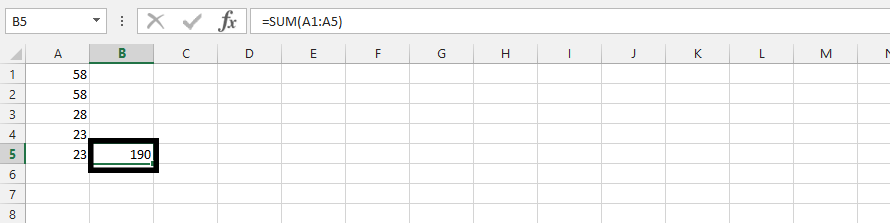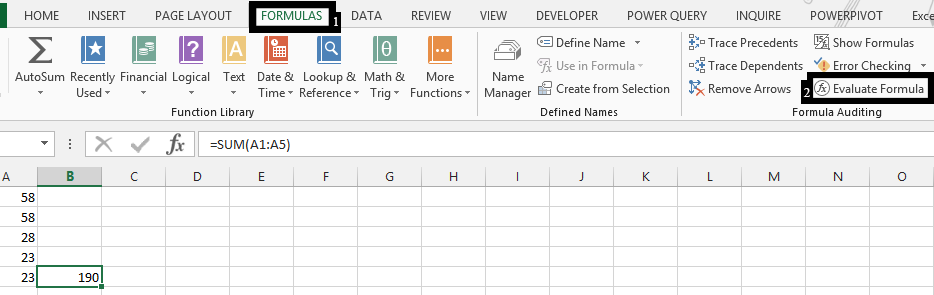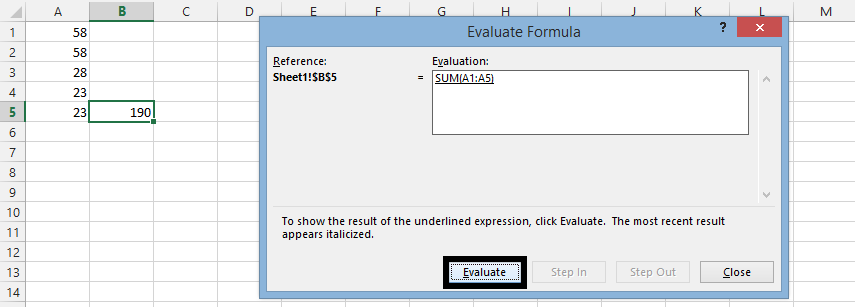#### How to Show Calculation Steps in Excel

To show calculation steps in Excel, you can use the “Evaluate Formula” feature. Here’s how to use it:

## Data preparation

Please see this data that shows how to show the formula calculation steps when there is an error.Note: It is clicking on the arrow (1) and then choosing “Show calculation steps” (2). This is perfect when the result has errors.

1. Select the result by clicking on it.## Showing formula calculation steps

2. Go to the “Formulas” tab and click on “Evaluate Formula”.3. The “Evaluate Formula” dialog box will appear, displaying the individual calculation steps. Click “Evaluate” to see the result of each calculation step.Keep clicking “Evaluation” to evaluate all your formulas. This is in case you have multiple formulas like =SUM(A2:A6,B2:B5). A2 to A6 is one formula, and B2 to B5 is another. Repeating this click on evaluate will show the result of A2:A6, and B2:B5 respectively.

This feature is particularly useful when you encounter errors or want to gain a deeper understanding of how a formula works.